Residue

Residue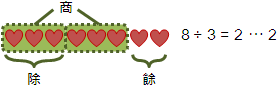```dividend   被除數
divisor    除數
quotient   商數
remainder  餘數
modulo     模數
residue    留數→餘數
congruence 全等→同餘
```

residue是數值。congruence是運算符號。

Residue (Sum)

modulo mod```8 (mod 3) + 7 (mod 3) ≡ 15 (mod 3)
8 (mod 3) + 7 (mod 3) + 6 (mod 3) ≡ 21 (mod 3)
```

```8 + 7 ≡ 15 (mod 3)
8 + 7 + 6 ≡ 21 (mod 3)
```

```  1 (mod 4) + 1 (mod 6)
≡ ⋯ ∪
{ 1 (mod 4) + -5 } ∪
{ 1 (mod 4) +  1 } ∪
{ 1 (mod 4) +  7 } ∪
⋯
≡ { 0 (mod 4) } ∪ { 2 (mod 4) }
```

subtraction −

```8 - 7 ≡ 1 (mod 3)
8 - 7 - 6 ≡ -5 (mod 3)
```

UVa 10787

multiplication ×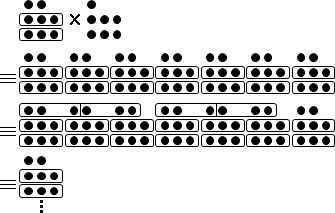```8 (mod 5) × 7 ≡ 56 (mod 5)
8 (mod 5) × 7 × 6 ≡ 336 (mod 5)
```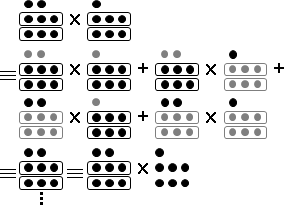```8 (mod 5) × 7 (mod 5) ≡ 56 (mod 5)
8 (mod 5) × 7 (mod 5) × 6 (mod 5) ≡ 336 (mod 5)
```

```8 × 7 ≡ 56 (mod 5)
8 × 7 × 6 ≡ 336 (mod 5)
```

exponentiation ^

```8 (mod 3) ^ 3 ≡ 8 (mod 3) × 8 (mod 3) × 8 (mod 3) ≡ 512 (mod 3)
```

```模數是質數p，次方值的模數正是p-1。根據「費馬小定理」。
2 (mod 7) ^ 100 ≡ 2 (mod 7) ^ 100 (mod 6)

2 (mod 9) ^ 100 ≡ 2 (mod 9) ^ 100 (mod λ(9))

φ(m)因數種類更多、內含錯誤，但是φ(m)比較容易計算。
2 (mod 9) ^ 100 ≡ 2 (mod 9) ^ 100 (mod φ(9))

```

```預先知道 φ(9) = 6
2 (mod 9) ^ 100
≡ 2 (mod 9) ^ 100 (mod φ(9))
≡ 2 (mod 9) ^ 100 (mod 6)
≡ 2 (mod 9) ^ 4 (mod 6)
≡ 16 (mod 9)
≡ 7 (mod 9)
```

UVa 374 luogu P1226

Residue (Fraction)

reciprocal ¹⁄ₓ

```☐ × 7 ≡ 1 (mod 5)
```

```1 ÷ 7 ≡ ☐ (mod 5)   除法風格
¹⁄₇   ≡ ☐ (mod 5)   分數風格
7⁻¹   ≡ ☐ (mod 5)   次方風格
7     ≡ ☐ (mod 5)   共軛風格
```

```☐ × 7 ≡ 1 (mod 5)   —→  1 ÷ 7 ≡ ☐ (mod 5)   ☐是3
3 × ☐ ≡ 1 (mod 4)   —→  1 ÷ 3 ≡ ☐ (mod 4)   ☐是3
2 × ☐ ≡ 1 (mod 4)   —→  1 ÷ 2 ≡ ☐ (mod 4)   ☐不存在
1 × ☐ ≡ 1 (mod 4)   —→  1 ÷ 1 ≡ ☐ (mod 4)   ☐是1
0 × ☐ ≡ 1 (mod 4)   —→  1 ÷ 0 ≡ ☐ (mod 4)   ☐不存在
```

```倒數的定義
a × a ≡ 1 (mod m)

a × a + m × k = 1
```

a與m的最大公因數必須是一（互質），才擁有倒數。

luogu P1082

reciprocal table ¹⁄₁ ... ¹⁄ₓ（模數是質數）

``` p % i = p - floor(p ÷ i) × i
p % i ≡   - floor(p ÷ i) × i          (mod p)
rec[i] ≡   - floor(p ÷ i) × rec[p % i] (mod p)   移項
```

reciprocal ¹⁄ₓ（模數是質數）

```a ^ p     ≡ a (mod p)
a ^ (p-1) ≡ 1 (mod p)   when a ≢ 0 (mod p)
a ^ (p-2) ≡ a (mod p)   when a ≢ 0 (mod p)
```

division ÷

```☐ × 7 ≡ 22 (mod 5)
```

```22 ÷ 7 ≡ ☐ (mod 5)
```

```☐ × 7 ≡ 22 (mod 5)  —→  22 ÷ 7 ≡ ☐ (mod 5)  ☐是1
☐ × 2 ≡ 3 (mod 4)   —→  3 ÷ 2 ≡ ☐ (mod 4)   ☐不存在
☐ × 4 ≡ 5 (mod 10)  —→  5 ÷ 4 ≡ ☐ (mod 10)  ☐不存在
☐ × 3 ≡ 0 (mod 6)   —→  0 ÷ 3 ≡ ☐ (mod 6)   ☐是0或2
☐ × 3 ≡ 3 (mod 12)  —→  3 ÷ 3 ≡ ☐ (mod 12)  ☐是1或5或9
```

```☐ × 7      ≡ 22     (mod 5)   求乘數
☐ × 7 × 7  ≡ 22 × 7 (mod 5)   等號兩側同乘以「7的倒數」
☐          ≡ 22 × 7 (mod 5)   「7」乘以「7的倒數」令它是1，就能消掉。
22 ÷ 7 ≡ ☐ ≡ 22 × 7 (mod 5)   「除以7」變成「乘以7的倒數」
```

```7 ≡ 3 (mod 5)   模5的時候，7的倒數是3。因為 7 × 3 ≡ 1 (mod 5)。

22 ÷ 7 ≡ 22 × 7 ≡ 22 × 3 ≡ 66 ≡ 1 (mod 5)
3 ÷ 7 ≡  3 × 7 ≡  3 × 3 ≡  9 ≡ 4 (mod 5)
2 ÷ 7 ≡  2 × 7 ≡  2 × 3 ≡  6 ≡ 1 (mod 5)
1 ÷ 7 ≡  1 × 7 ≡  1 × 3 ≡  3 ≡ 3 (mod 5)
```

```a   ÷ b   ≡ ☐ (mod m)
a/d ÷ b/d ≡ ☐ (mod m/d)
```

luogu P2613

linear congruence ax ≡ b (mod m)

Residue (Fractions)

monic linear congruences x ≡ rᵢ (mod mᵢ) , mᵢ are coprime

```{ x ≡ 2 (mod 3)
{ x ≡ 3 (mod 5)
{ x ≡ 2 (mod 7)
```

```x ≡ 23 (mod 105)
```

```{ x ≡ 2 (mod 3)  ⇔  x = ..., 8, 5, 2, -1, -4, ...
{ x ≡ 3 (mod 5)  ⇔  x = ..., 13, 8, 3, -2, -7, ...
{ x ≡ 2 (mod 7)  ⇔  x = ..., 16, 9, 2, -5, -12, ...
```

```{ x ≡ 2 (mod 3)
{ x ≡ 3 (mod 5)
{ x ≡ 2 (mod 7)

⇒ x = 3t + 2

⇒ 3t + 2 ≡ 3 (mod 5) ⇒ 3t ≡ 1 (mod 5) ⇒ t ≡ 2 (mod 5)
⇒ t = 5s + 2

⇒ x = 3(5s + 2) + 8 = 15s + 8

⇒ 15s + 8 ≡ 2 (mod 7) ⇒ 15s ≡ 1 (mod 7) ⇒ s ≡ 1 (mod 7)
⇒ s = 7u + 1

⇒ x = 15(7u + 1) + 8 = 105u + 23
```

```一、一元一次方程組，係數皆是一，模數 m₁ m₂ 互質。
{ x ≡ r₁ (mod m₁)
{ x ≡ r₂ (mod m₂)

x ≡ r₁ + k m₁ (mod m₁)

x ≡ r₁ + k m₁ ≡ r₂           (mod m₂)
k m₁ ≡ r₂ - r₁      (mod m₂)
k    ≡ (r₂ - r₁) m₁ (mod m₂)

{ x ≡ r₁ + k m₁ (mod m₁)     where k ≡ (r₂ - r₁) m₁ (mod m₂)
{ x ≡ r₁ + k m₁ (mod m₂)

x ≡ r₁ + k m₁ (mod m₁m₂)     where k ≡ (r₂ - r₁) m₁ (mod m₂)
```

```一、一元一次方程組，係數皆是一，模數 m₁ 到 mN 兩兩互質。
{ x ≡ r₁ (mod m₁)
{ ⋮
{ x ≡ rN (mod mN)

M₁ = M ÷ m₁
⋮
MN = M ÷ mN

Mᵢ 原本包含所有模數，但是刻意消去了 mᵢ。
造成 Mᵢ 只對其中一個模數 mᵢ 有反應（無法整除）（互質）：
Mᵢ % mⱼ ≠ 0   when i = j
Mᵢ % mⱼ = 0   when i ≠ j

⋮
MN = MN的倒數，模數為 mN

讓 (Mᵢ × Mᵢ) 只對其中一個模數 mᵢ 有反應，得到結果是 1：
(Mᵢ × Mᵢ) % mⱼ = 1   when i = j
(Mᵢ × Mᵢ) % mⱼ = 0   when i ≠ j

再讓 (rᵢ × Mᵢ × Mᵢ) 只對其中一個模數 mᵢ 有反應，得到結果是 rᵢ。
(rᵢ × Mᵢ × Mᵢ) % mⱼ = rᵢ   when i = j
(rᵢ × Mᵢ × Mᵢ) % mⱼ = 0    when i ≠ j

x ≡ (r₁ × M₁ × M₁) (mod m₁) + ... + (rN × MN × MN) (mod mN)

x ≡ (r₁ × M₁ × M₁) + ... + (rN × MN × MN) (mod M)
```

monic linear congruences x ≡ rᵢ (mod mᵢ)

```                     { x ≡ 4 (mod 9)
x ≡ 4 (mod 360)  —→  { x ≡ 4 (mod 10)
{ x ≡ 4 (mod 24)

where 360 = lcm(9, 10, 24)
```

```                     { x ≡ 4 (mod 2³)
x ≡ 4 (mod 360)  —→  { x ≡ 4 (mod 3²)
{ x ≡ 4 (mod 5¹)

where 360 = 2³ × 3² × 5¹ = lcm(2³, 3², 5¹)
```

```                      { x ≡ 2 (mod 2²)✔ 10₍₂₎
{ x ≡ 2 (mod 4)       { x ≡ 6 (mod 3¹)
{ x ≡ 6 (mod 48)  —→  { x ≡ 6 (mod 2⁴)✔110₍₂₎
{ x ≡ 4 (mod 360)     { x ≡ 4 (mod 2³)✔100₍₂₎
{ x ≡ 4 (mod 3²)   ↑
{ x ≡ 4 (mod 5¹)   no solution
```
```                      { x ≡ 1 (mod 2²)✔  1₍₂₎
{ x ≡ 1 (mod 4)       { x ≡ 7 (mod 3¹)             { x ≡ 7 (mod 2⁴)
{ x ≡ 7 (mod 48)  —→  { x ≡ 7 (mod 2⁴)✔111₍₂₎  —→  { x ≡ 1 (mod 3²)
{ x ≡ 1 (mod 360)     { x ≡ 1 (mod 2³)✔  1₍₂₎      { x ≡ 1 (mod 5¹)
{ x ≡ 1 (mod 3²)
{ x ≡ 1 (mod 5¹)
```

luogu P4777

linear congruences aᵢx ≡ bᵢ (mod mᵢ)

```{ 1x ≡ 2 (mod 3)      { x ≡ 1×2 (mod 3)      { x ≡ 2 (mod 3)
{ 3x ≡ 3 (mod 5)  —→  { x ≡ 3×3 (mod 5)  —→  { x ≡ 6 (mod 5)
{ 5x ≡ 2 (mod 7)      { x ≡ 5×2 (mod 7)      { x ≡ 6 (mod 7)
```

Chinese Remainder Theorem

```一元一次方程組、係數為一、模數互質
{ r ≡ r₁ (mod m₁)
{ r ≡ r₂ (mod m₂)   where mᵢ⊥mⱼ
{ :   :    :  :

r (mod m₁m₂...)  ←—→  (r₁ (mod m₁), r₂ (mod m₂), ...)

(m₁, m₂, ...)
r  ←—————————————→  (r₁, r₂, ...)
```
```一元一次方程組、係數為一、模數互質
{ 10 ≡ 1 (mod 3)
{ 10 ≡ 0 (mod 5)
{ 10 ≡ 3 (mod 7)

10 (mod 3×5×7)  ←—→  (1 (mod 3), 0 (mod 5), 3 (mod 7))

(3,5,7)
10  ←———————→  (1,0,3)
```
```    已知一組模數，分別計算餘數。O(N)
r  —————————————————————————————————→  (r₁, r₂, ...)
←—————————————————————————————————
解方程組。O(Nlog(m))
```

```分解前  模數 105 | 分解後                     模數 (3, 5, 7)
5                | (2, 0, 5)
9                | (0, 4, 2)
-----------------+------------------------------------------
5 + 9 ≡ 14       | (2+0, 0+4, 5+2) ≡ (2, 4, 7)
5 - 9 ≡ -4 ≡ 101 | (2-0, 0-4, 5-2) ≡ (2, -4, 3) ≡ (2, 1, 3)
5 × 9 ≡ 45       | (2×0, 0×4, 5×2) ≡ (0, 0, 10)
5 ÷ 9 ≡ undefine | (2÷0, 0÷4, 5÷2) ≡ undefine
5 ÷ 8 ≡ 40       | (2÷2, 0÷3, 5÷1) ≡ (1, 0, 5)
5 ^ 2 ≡ 25       | (2^2, 0^2, 5^2) ≡ (4, 0, 25) ≡ (1, 0, 4)
```

UVa 756 700 11754

```(999 + 25) × 333 ÷ 256 = ?

{ (999 + 25) × 333 ÷ 256 ≡ (0 + 1) × 0 ÷ 1 ≡ 0 (mod 3)
{ (999 + 25) × 333 ÷ 256 ≡ (-1 + 0) × 3 ÷ 1 ≡ 2 (mod 5)
{ (999 + 25) × 333 ÷ 256 ≡ (9 + 3) × 3 × 4 ≡ 1 × 1 ≡ 1 (mod 11)

=> (999 + 25) × 333 ÷ 256 ≡ 12 (mod 165)

999+25是4位數，333是3位數，256是3位數，推測正確答案是4位數：
12 + 165 × 6 = 1002
1002 + 165 = 1167
1167 + 165 = 1332
......
```

Residue (Logarithm)

multiplicative order ord()

```2 ^ ☐ ≡ 1 (mod 9)
```

```ord₉(2) = ☐
```

```0 ^ ☐ ≡ 1 (mod 9)  —→  ord₉(0) = ☐   ☐是0            	→未定義
1 ^ ☐ ≡ 1 (mod 9)  —→  ord₉(1) = ☐   ☐是0...8        	→只取1
2 ^ ☐ ≡ 1 (mod 9)  —→  ord₉(2) = ☐   ☐是0或6         	→只取6
3 ^ ☐ ≡ 1 (mod 9)  —→  ord₉(3) = ☐   ☐是0            	→未定義
4 ^ ☐ ≡ 1 (mod 9)  —→  ord₉(4) = ☐   ☐是0或3或6      	→只取3
5 ^ ☐ ≡ 1 (mod 9)  —→  ord₉(5) = ☐   ☐是0或6         	→只取6
6 ^ ☐ ≡ 1 (mod 9)  —→  ord₉(6) = ☐   ☐是0            	→未定義
7 ^ ☐ ≡ 1 (mod 9)  —→  ord₉(7) = ☐   ☐是0或3或6      	→只取3
8 ^ ☐ ≡ 1 (mod 9)  —→  ord₉(8) = ☐   ☐是0或2或4或6或8	→只取2
```

discrete logarithm log() / index ind()

```2 ^ ☐ ≡ 7 (mod 9)
```

```ind₂(7 (mod 9)) ≡ ☐ (mod ord₉(2))
```

UVa 10225 11916 luogu P4195

```a ^ x ≡ b (mod m)   已知a b m，求x。另外a ⊥ m。

甲、計算a⁰, a¹, ..., aⁿ⁻¹，
如果等於b，就找到答案了。

甲、一口氣處理aⁿ到a²ⁿ⁻¹：
aⁿ⁺ⁱ ≡ b
aⁿ × aⁱ ≡ b
aⁱ ≡ b × rec(aⁿ)
先前計算的a⁰, a¹, ..., aⁿ⁻¹，
如果等於b × rec(aⁿ)，就找到答案了。
乙、一口氣處理a²ⁿ到a³ⁿ⁻¹，
a²ⁿ⁺ⁱ ≡ b
aⁿ × aⁿ × aⁱ ≡ b
aⁱ ≡ b × rec(aⁿ) × rec(aⁿ)
先前計算的a⁰, a¹, ..., aⁿ⁻¹，
如果等於b × rec(aⁿ) × rec(aⁿ)，就找到答案了。
丙、如法炮製。
```

```二、Baby-step，一步一個數字，總共n步，第一區塊：
甲、處理a⁰到aⁿ⁻¹：
aⁱ ≡ b
aⁿ ≡ b × aⁿ⁻ⁱ
計算baⁿ, ..., ba², ba¹，
如果等於aⁿ，就找到答案了。

甲、一口氣處理aⁿ到a²ⁿ⁻¹：
aⁿ⁺ⁱ ≡ b
a²ⁿ  ≡ b × aⁿ⁻ⁱ
先前計算的baⁿ, ..., ba², ba¹，
如果等於a²ⁿ，就找到答案了。
乙、如法炮製。
```

Residue (Root)

root of unity n1

```☐ ^ 3 ≡ 1 (mod 9)
```

```∛1 ≡ ☐ (mod 9)
```

nth root n

```☐ ^ 3 ≡ 7 (mod 9)
```

```∛7 ≡ ☐ (mod 9)
```

ICPC 4746 luogu P5668

square root √‾

```☐ ^ 2 ≡ 7 (mod 9)
```

```√7 ≡ ☐ (mod 9)
```

UVa 10831 luogu P5491 U124195

```http://library.msri.org/books/Book44/files/02buhler.pdf
solve y² ≡ c (mod p)
(1) select random number b such that D = b² - 4c is non-residue
(2) α is a root of (x² - bx + c)
thus β = α^p is also a root
thus c = αβ = α^(p+1)
(3) return α^((p+1)/2)
```
```https://arxiv.org/abs/1309.2831
solve y² ≡ c (mod p)
(1) h := (b² - 4c)^((p-1)/2) (mod p)
(2) if h ≡ 0 or 1 (mod p) then s := 0
(3) if h ≡ -1 (mod p) then s := 1
(4) q(x) := x^((p+1)/2) mod (x² - bx + c)
where q(x) = c₁x + c₀ for integers c₀, c₁
(5) y := s c₀
(6) Return y as the output
```

Residue (Primitive Root)

primitive root【查無數學運算符號】

```質因數分解φ(m)，得到質因數p₁ p₂ ...。

（φ(m)改成λ(m)，檢測次數更少；然而λ(m)更難算。）
```

luogu P6091

```4^5與8^3誰比較大？

4^5 = (2^2)^5 = 2^10
8^3 = (2^3)^3 = 2^9
```
```x ^ a ≡ b (mod m)   已知a b m，求x。

```

Residue (Group)

```實數系統的加法表、乘法表
+ | 0  1  2  3 ...   × | 0  1  2  3 ...
--|---------------   --|---------------
0 | 0  1  2  3 ...   0 | 0  0  0  0 ...
1 | 1  2  3  4 ...   1 | 0  1  2  3 ...
2 | 2  3  4  5 ...   2 | 0  2  4  6 ...
3 | 3  4  5  6 ...   3 | 0  3  6  9 ...
: | :  :  :  :       : | :  :  :  :
```
```餘數系統的加法表、乘法表
(mod 1)  (mod 2)    (mod 3)       (mod 4)          (mod 5)
+ | 0    + | 0  1   + | 0  1  2   + | 0  1  2  3   + | 0  1  2  3  4
--|--    --|-----   --|--------   --|-----------   --|--------------
0 | 0    0 | 0  1   0 | 0  1  2   0 | 0  1  2  3   0 | 0  1  2  3  4
1 | 1  0   1 | 1  2  0   1 | 1  2  3  0   1 | 1  2  3  4  0
2 | 2  0  1   2 | 2  3  0  1   2 | 2  3  4  0  1
3 | 3  0  1  2   3 | 3  4  0  1  2
4 | 4  0  1  2  3

(mod 1)  (mod 2)    (mod 3)       (mod 4)          (mod 5)
× | 0    × | 0  1   × | 0  1  2   × | 0  1  2  3   × | 0  1  2  3  4
--|--    --|-----   --|--------   --|-----------   --|--------------
0 | 0    0 | 0  0   0 | 0  0  0   0 | 0  0  0  0   0 | 0  0  0  0  0
1 | 0  1   1 | 0  1  2   1 | 0  1  2  3   1 | 0  1  2  3  4
2 | 0  2  1   2 | 0  2  0  2   2 | 0  2  4  1  3
3 | 0  3  2  1   3 | 0  3  1  4  2
4 | 0  4  3  2  1
```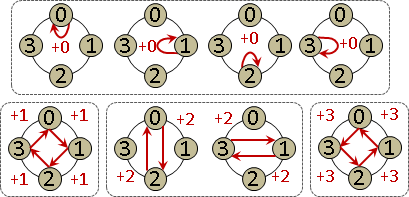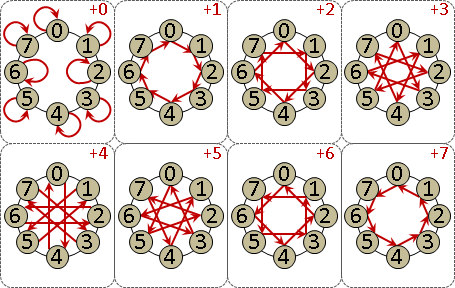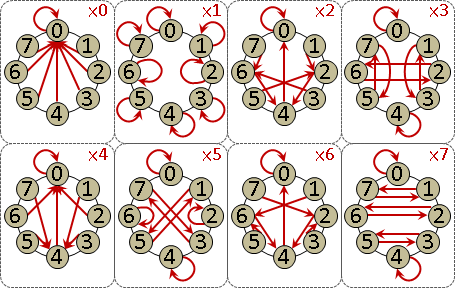「與模數互質的數字」不斷相乘，絕不會得到「不與模數互質的數字」，而且所有數字都位於某個循環節之內。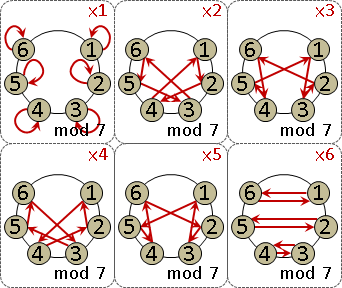「與模數互質的數字」暨乘法除法，性質優美，自成一格，數學家稱作「乘法群」。一種模數得到一種乘法群。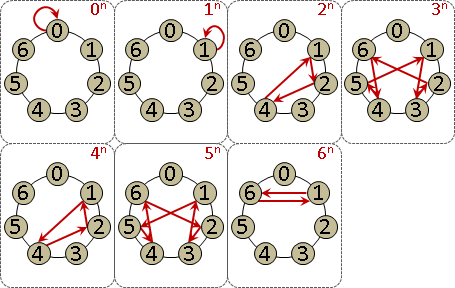2¹ 2⁵ 2⁷ 2¹¹分別等於2 6 11 7。得到模數13的所有原根。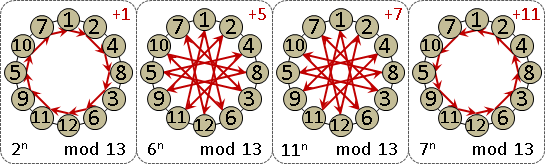Residue (Polynomial)

polynomial n-1i=0cᵢxⁱ

```x³ + 2x² - 5x + 1 (mod 3)
```

```x³ + 2x² - 5x + 1 ≡ x³ + 2x² + x + 1 (mod 3)
```

factorization n = ω(n)k=1pₖeₖ

```列舉質數：2, 3, 5, 7, 11, ...

```

```列舉質數：2 (mod 8), 3 (mod 8), ...  ✘

```

polynomial factorization f(x) = deg(f)i=0(x - aᵢ)

```列舉質式：x, x + 1, x + 2, ...

```

```列舉質式：x (mod 2), x + 1 (mod 2), x² + x + 1 (mod 2), ...

```

polynomial congruence f(x) ≡ b (mod m)

```x² - 2x + 1 ≡ 3x² + x + 1 (mod 5)
```

```x³ + 2x² - 5x + 1 ≡ 0 (mod 3)
```

```x ≡ 1 (mod 3)
```

```Cantor–Zassenhaus(f)
{
f = gcd(f, x^p - x)
if (deg(f) == 1) return root(f)
while (true)
{
b = random()
g = gcd(f, (x+b)^((p-1)/2) - 1)
if (0 < deg(g) < deg(f)) return CZ(g) ∪ CZ(f/g)
}
}
```

Hensel Lifting將模數從質數拓展至質數次方。

```given f(a) ≡ 0 (mod pⁿ)
find f(aꜛ) ≡ 0 (mod p²ⁿ)
assume aꜛ ≡ a (mod pⁿ)
f(aꜛ) ≡ f(a)(aꜛ-a)⁰ + f′(a)(aꜛ-a)¹ + f″(a)(aꜛ-a)²/2! + ... (mod p²ⁿ)
f(aꜛ) ≡ f(a) + f′(a)(aꜛ-a) (mod p²ⁿ)      ^^^^^^^ double the zeros
aꜛ ≡ a - f(a)​ / f′(a) (mod p²ⁿ)
aꜛ ≡ a - f(a)​ / f′(a) (mod pⁿ⁺¹)   weak version
```

```{ x ≡ a₁ (mod p₁e₁)
{ x ≡ a₂ (mod p₂e₂)  ←—→  x ≡ a (mod p₁e₁ × p₂e₂ × ...)
{ :   :       :
```

linear congruences Ax⃗ ≡ b⃗ (mod m)

```{  x - 2y + 1z ≡ 1 (mod m)
{ 3x + 2y + 1z ≡ 2 (mod m)
{  x + 2y + 3z ≡ 3 (mod m)
```

luogu P4783

system of congruences F(x⃗) ≡ y⃗ (mod m⃗)

```{  x² - 2x + 1 ≡ x + 1  (mod 3)
{ 3x² + 2x + 1 ≡ x - 1  (mod 5)
{  x² + 2x + 3 ≡ x² + 1 (mod 7)
```

Pendulum Snake

Faro Shuffle

Water Jug Problem

BFS Tree恰好是水量變化最少的路線。可用下面的模型證明。

UVa 571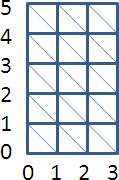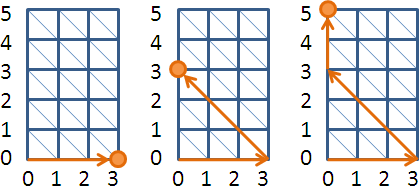ICPC 6501

Josephus Problem

UVa 133 305 402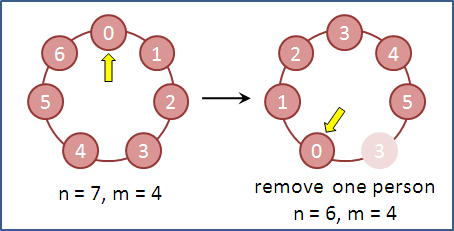```f(n, m) = (f(n-1, m) + m) % n
f(1, m) = 0;

f(n, m)：最後活下來的人的編號。
```

UVa 10015 151 440 10940 11351 180